moveit2 The MoveIt Motion Planning Framework for ROS 2.
ompl_interface::OrientationConstraint Class Reference

Orientation constraints parameterized using exponential coordinates. More...

`#include <ompl_constraints.h>`

Inheritance diagram for ompl_interface::OrientationConstraint: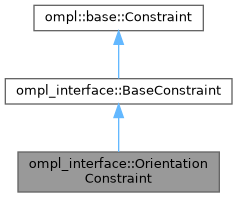[legend]
Collaboration diagram for ompl_interface::OrientationConstraint: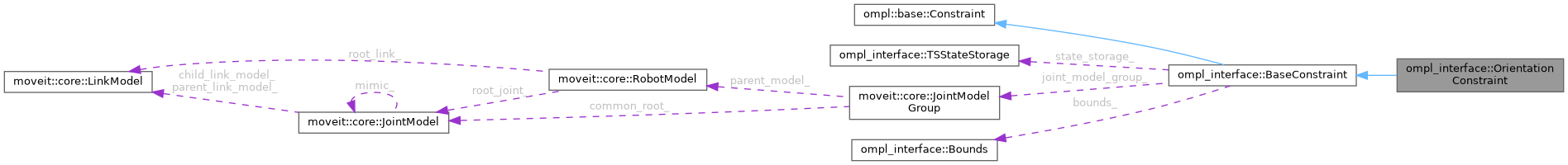[legend]

## Public Member Functions

OrientationConstraint (const moveit::core::RobotModelConstPtr &robot_model, const std::string &group, const unsigned int num_dofs)

void parseConstraintMsg (const moveit_msgs::msg::Constraints &constraints) override
Parse bounds on orientation parameters from MoveIt's constraint message. More...

Eigen::VectorXd calcError (const Eigen::Ref< const Eigen::VectorXd > &x) const override
For inequality constraints: calculate the value of the parameter that is being constrained by the bounds. More...

Eigen::MatrixXd calcErrorJacobian (const Eigen::Ref< const Eigen::VectorXd > &x) const override
For inequality constraints: calculate the Jacobian for the current parameters that are being constrained.

• This error jacobian, as the name suggests, is only the jacobian of the position / orientation / ... error. It does not take into account the derivative of the penalty functions defined in the Bounds class. This correction is added in the implementation of of BaseConstraint::jacobian.
More...Public Member Functions inherited from ompl_interface::BaseConstraint
BaseConstraint (const moveit::core::RobotModelConstPtr &robot_model, const std::string &group, const unsigned int num_dofs, const unsigned int num_cons=3)
Construct a BaseConstraint using 3 `num_cons` by default because all constraints currently implemented have 3 constraint equations. More...

void init (const moveit_msgs::msg::Constraints &constraints)
Initialize constraint based on message content. More...

void function (const Eigen::Ref< const Eigen::VectorXd > &joint_values, Eigen::Ref< Eigen::VectorXd > out) const override

void jacobian (const Eigen::Ref< const Eigen::VectorXd > &joint_values, Eigen::Ref< Eigen::MatrixXd > out) const override
Jacobian of the constraint function. More...

Eigen::Isometry3d forwardKinematics (const Eigen::Ref< const Eigen::VectorXd > &joint_values) const
Wrapper for forward kinematics calculated by MoveIt's Robot State. More...

Eigen::MatrixXd robotGeometricJacobian (const Eigen::Ref< const Eigen::VectorXd > &joint_values) const
Calculate the robot's geometric Jacobian using MoveIt's Robot State. More...

const Eigen::Vector3d getTargetPosition ()

const Eigen::Quaterniond getTargetOrientation ()Protected Attributes inherited from ompl_interface::BaseConstraint
TSStateStorage state_storage_
Thread-safe storage of the robot state. More...

const moveit::core::JointModelGroupjoint_model_group_

Robot link the constraints are applied to. More...

Bounds bounds_
Upper and lower bounds on constrained variables. More...

Eigen::Vector3d target_position_
target for equality constraints, nominal value for inequality constraints. More...

Eigen::Quaterniond target_orientation_
target for equality constraints, nominal value for inequality constraints. More...

## Detailed Description

Orientation constraints parameterized using exponential coordinates.

An orientation constraint is modeled as a deviation from a target orientation. The deviation is represented using exponential coordinates. A three element vector represents the rotation axis multiplied with the angle in radians around this axis.

R_error = R_end_effector ^ (-1) * R_target R_error -> rotation angle and axis (using Eigen3) error = angle * axis (vector with three elements)

And then the constraints can be written as

• absolute_x_axis_tolerance < error < absolute_x_axis_tolerance
• absolute_y_axis_tolerance < error < absolute_y_axis_tolerance
• absolute_z_axis_tolerance < error < absolute_z_axis_tolerance

IMPORTANT It is NOT how orientation error is handled in the default MoveIt constraint samplers, where XYZ intrinsic euler angles are used. Using exponential coordinates is analog to how orientation error is calculated in the TrajOpt motion planner.

Definition at line 377 of file ompl_constraints.h.

## ◆ OrientationConstraint()

 ompl_interface::OrientationConstraint::OrientationConstraint ( const moveit::core::RobotModelConstPtr & robot_model, const std::string & group, const unsigned int num_dofs )
inline

Definition at line 380 of file ompl_constraints.h.

## ◆ calcError()

 Eigen::VectorXd ompl_interface::OrientationConstraint::calcError ( const Eigen::Ref< const Eigen::VectorXd > & x ) const
overridevirtual

For inequality constraints: calculate the value of the parameter that is being constrained by the bounds.

In this orientation constraints case, it calculates the orientation of the end-effector. This error is then converted in generic equality constraints in the implementation of `ompl_interface::BaseConstraint::function`.

This method can be bypassed if you want to override `ompl_interface::BaseConstraint::function directly and ignore the bounds calculation.

Reimplemented from ompl_interface::BaseConstraint.

Definition at line 312 of file ompl_constraints.cpp.

Here is the call graph for this function: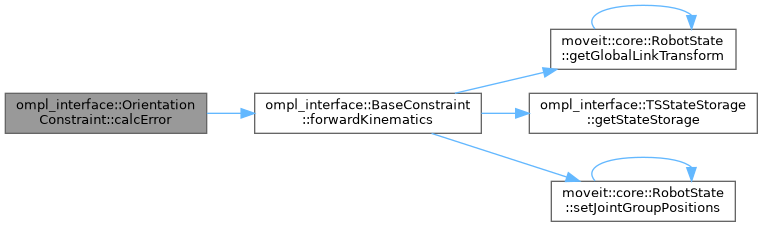## ◆ calcErrorJacobian()

 Eigen::MatrixXd ompl_interface::OrientationConstraint::calcErrorJacobian ( const Eigen::Ref< const Eigen::VectorXd > & x ) const
overridevirtual

For inequality constraints: calculate the Jacobian for the current parameters that are being constrained.

• This error jacobian, as the name suggests, is only the jacobian of the position / orientation / ... error. It does not take into account the derivative of the penalty functions defined in the Bounds class. This correction is added in the implementation of of BaseConstraint::jacobian.

This method can be bypassed if you want to override `ompl_interface::BaseConstraint::jacobian directly and ignore the bounds calculation.

TODO(jeroendm), Maybe also use an output argument as in `ompl::base::Constraint::jacobian(x, out)` for better performance?

Reimplemented from ompl_interface::BaseConstraint.

Definition at line 319 of file ompl_constraints.cpp.

Here is the call graph for this function: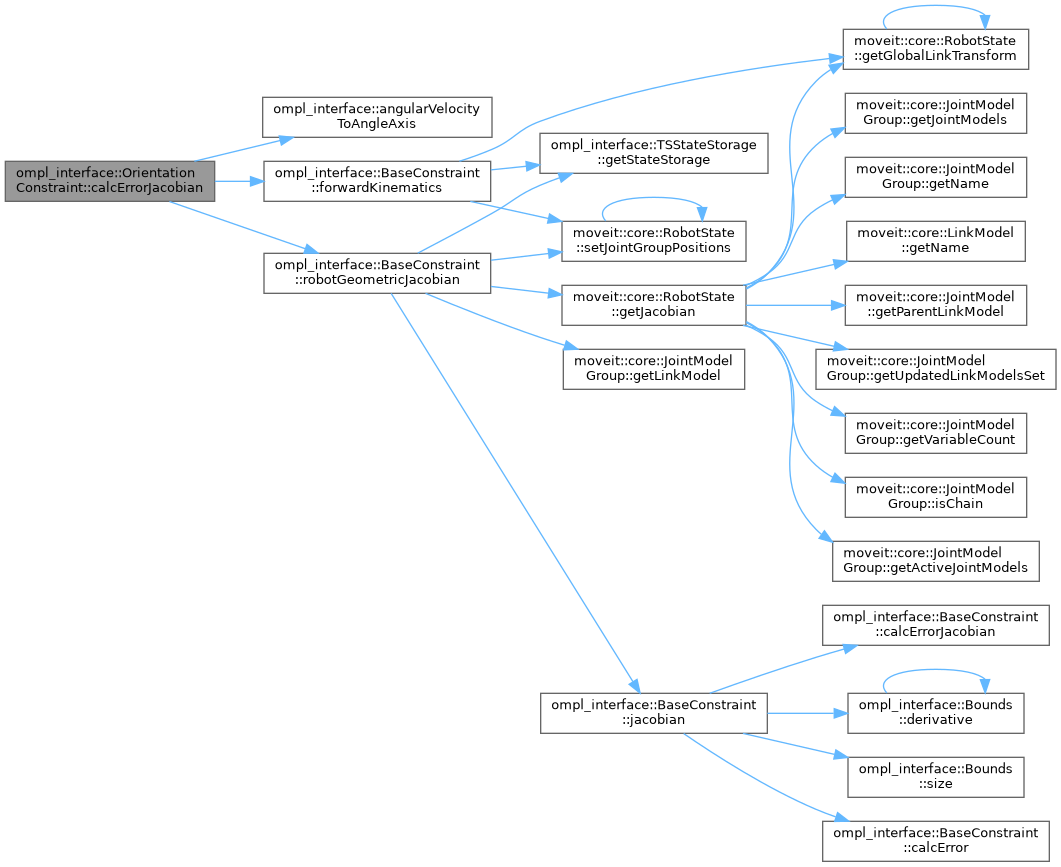## ◆ parseConstraintMsg()

 void ompl_interface::OrientationConstraint::parseConstraintMsg ( const moveit_msgs::msg::Constraints & constraints )
overridevirtual

Parse bounds on orientation parameters from MoveIt's constraint message.

This can be non-trivial given the often complex structure of these messages.

Implements ompl_interface::BaseConstraint.

Definition at line 303 of file ompl_constraints.cpp.

Here is the call graph for this function: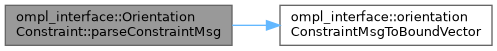The documentation for this class was generated from the following files: